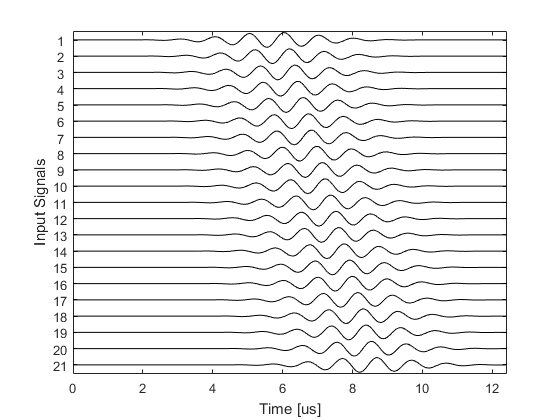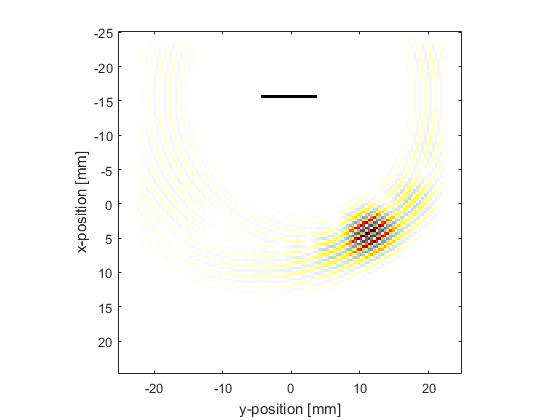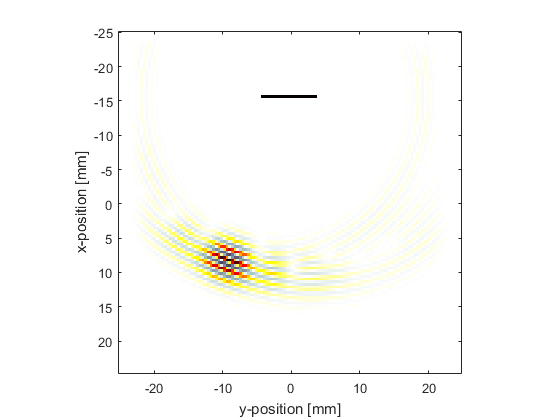# Steering A Linear Array Example

This example demonstrates how to use k-Wave to steer a tone burst from a linear array transducer in 2D. It builds on the Simulating Transducer Field Patterns Example.

## Defining the linear array and input signal

The source mask is defined by creating a binary mask with the desired source points set to 1.

```% define source mask for a linear transducer with an odd number of elements
num_elements = 21;      % [grid points]
x_offset = 25;          % [grid points]
start_index = Ny/2 - round(num_elements/2) + 1;
source.p_mask(x_offset, start_index:start_index + num_elements - 1) = 1;
```

The input signal to each element is then created using the function `toneBurst` with a geometrically steered temporal offset that varies across the source.

```% define the properties of the tone burst used to drive the transducer
sampling_freq = 1/kgrid.dt;     % [Hz]
steering_angle = 30;            % [deg]
element_spacing = dx;           % [m]
tone_burst_freq = 1e6;          % [Hz]
tone_burst_cycles = 8;

% create an element index relative to the centre element of the transducer
element_index = -(num_elements - 1)/2:(num_elements - 1)/2;

% use geometric beam forming to calculate the tone burst offsets for each
% transducer element based on the element index
tone_burst_offset = 40 + element_spacing * element_index * ...
sin(steering_angle * pi/180) / (medium.sound_speed * kgrid.dt);

% create the tone burst signals
source.p = toneBurst(sampling_freq, tone_burst_freq, tone_burst_cycles, ...
'SignalOffset', tone_burst_offset);
```

A plot of the input signals for each transducer element is shown below.## Running the simulation

Visualisations of the pressure field at two different times for steering angles of +30 and -20 degrees are shown below.